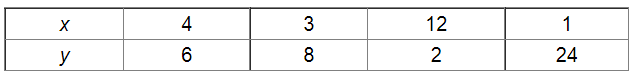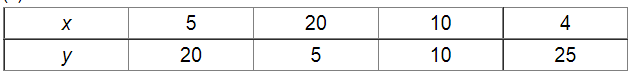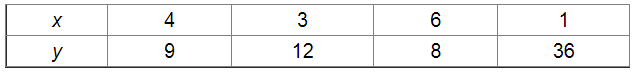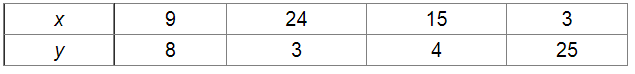# In which of the following tables x and y vary inversely:`
Question:

In which of the following tables x and y vary inversely:

(i)(ii)(iii)(iv)Solution:

(i) Since $x$ and $y \operatorname{var} y$ inversely, we have :

$y=\frac{k}{x}$

$\Rightarrow x y=k$

$\therefore$ The product of $x$ and $y$ is constant.

In all cases, the product $x y$ is constant $($ i.e., 24).

Thus, in this case, $x$ and $y$ var $y$ inversely.

(ii) In all ca $s$ es, the product $x y$ is constant for any two pairs of values for $x$ and $y$.

Here, $x y=100$ for all cases

Thus, in this case, $x$ and $y$ var $y$ inversely.

(iii) If $x$ and $y \operatorname{var} y$ inversely, the product $x y$ should be constant.

Here, in one case, product $=6 \times 8=48$ and in the rest, product $=36$

Thus, in this case, $x$ and $y$ do not var $y$ inversely.

(iv) If $x$ and $y$ var $y$ inversely, the produc $t x y$ should be constant.

Here, product is different for all cases.

Thus, in this case, $x$ and $y$ do not var $y$ inversely.